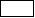# Boundaries of the study domain

## Introduction

The definition of a thermal field finite elements problem requires the input of:

• the computation (study) domain, i.e. to define the boundaries of the different regions where the temperature field is studied;
• the boundary conditions, i.e. to define the values of the state variable (temperature) on the computation domain boundaries.

## Boundaries of the computation domain

The study domain in thermal applications includes only the solid parts of the modeled device. The air regions are not included in the computation domain, and consequently, the infinite box region is not used to model the air. The boundary conditions on the boundaries of the study domain are set by using non-material boundary regions (see § Describing boundary conditions).

In the absence of non-material boundary regions, the default boundary condition is applied. This condition characterizes the adiabatic boundaries, where the thermal exchange is null 0 = 0).

## Symmetries and periodicities

If the geometry of the studied device exhibits symmetries, the heat flux density is tangent to the symmetry planes. It is not possible to define a heat flux density normal to the symmetry plane.

If the studied device exhibits periodicities , the user must enter the type of the periodicities (cyclic or anti-cyclic conditions) on the periodicity planes defined in the geometry context. An anti-cyclic periodicity condition has no physical meaning (it might allow negative temperatures in kelvin on the boundary).

## Typical example

A typical example of a thermal problem is presented in the figure below.: Regions representing the solid parts of the studied device (volume regions in 3D and surface regions in 2D): Regions representing the boundaries of the study domain (face regions in 3D and line regions in 2D)